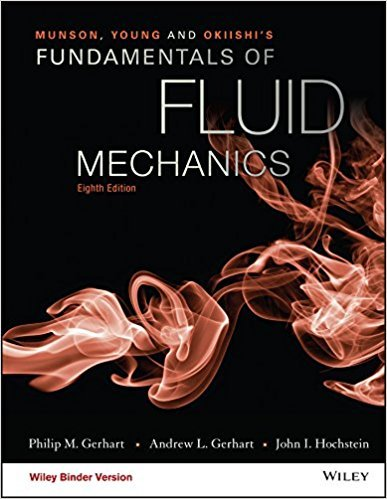# Air flows steadily between two sections in a duct. At section (1),the temperature and

Chapter 11, Problem 11.2

#### (choose chapter or problem)

Air flows steadily between two sections in a duct. At section (1),the temperature and pressure are T1 = 80 C, p1 = 301 kPa(abs),and at section (2), the temperature and pressure are T2 = 180 C,p2 = 181 kPa(abs). Calculate the (a) change in internal energybetween sections (1) and (2), (b) change in enthalpy between sections(1) and (2), (c) change in density between sections (1) and (2),(d) change in entropy between sections (1) and (2). How would youestimate the loss of available energy between the two sections ofthis flow?

Unfortunately, we don't have that question answered yet. But you can get it answered in just 5 hours by Logging in or Becoming a subscriber.